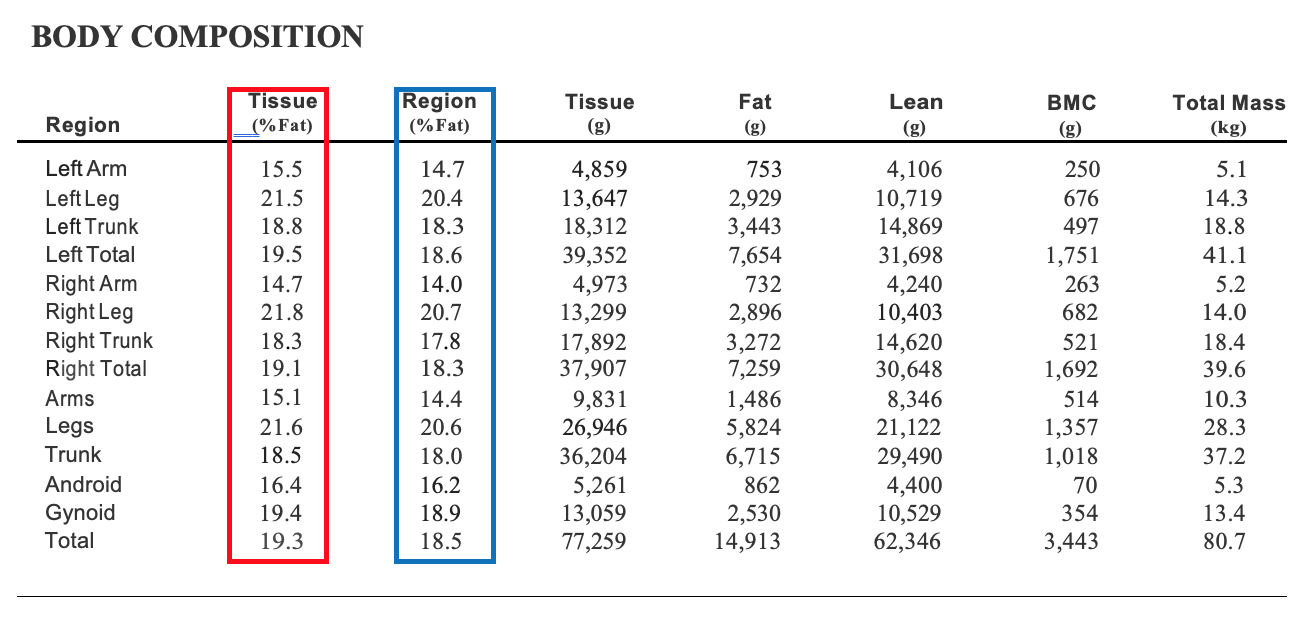If you own a GE iDXA or Prodigy X-ray absorptiometer (DXA) scanner you may notice on the body composition report that percent fat (%fat) is listed as Tissue (%fat) (red rectangle) and Region (%fat) (blue rectangle)(see example below).  I am usually asked two questions concerning these two values. The first question is what is the difference between Tissue %fat and Region %fat? The second question is which one should I report?  In this blog post, I try to answer both of these questions.So let us start with the first question.  What is the difference between Tissue %fat and Region %fat?  The Tissue %fat value is determined by dividing fat mass by tissue mass. The product of that division is then multiplied by 100.  For example, the total fat mass for this individual is 14,913 grams.  Divide the total fat mass by the total tissue mass (77,259 grams) and you get 0.19303. Since %fat is a percentage you multiply this by 100 and you get 19.3 %fat. A follow-up question would be what is total tissue mass? Total tissue mass is total fat mass plus total lean mass. Therefore, in the example, you add the fat mass (14,913 grams) to the lean mass (63,346 grams) and you get a tissue mass of 77,235 grams.  You may have noticed that bone mineral content (BMC) or bone mass is not included in the tissue mass. Obviously, that makes for a lower value for total tissue mass. Region %fat on the other hand is determined by taking the fat mass and dividing it by the total mass of the body. To get total mass you simply add total fat mass (14,913 grams), total lean mass (62,346 grams), and total BMC (3,443 grams) together. Therefore, for the example provide in this blog post, the total mass is 80,678 grams or 80.678 kilograms. You will notice that this matches with the total mass (80.7 kilograms). Therefore, the Region %fat is the fat mass (14,913 grams) divided by the total mass (80,678 grams) multiplied by 100. Performing that calculation, you end up with a Region %fat of 18.5%.

This leads us to the second question, which value should I report to the subject.  Most people tend to report the Region %fat. You may ask why. One simple, unscientific reason is that in most individuals Region %fat is lower than the Tissue %fat. Therefore reporting this value makes the subject feel better about their body composition. The more scientific reason is that Region %fat takes into account the entire mass of the body and not just the soft tissue mass. Finally, when %fat is determined by two-compartment methods of body composition (i.e., bioelectrical impedance analysis, hydrostatic weighing, skinfolds, etc.) bone mass is always included in the calculation of %fat. Therefore, if you want to compare across the methods you need to use Region %fat instead of Tissue %fat.

Take-Home Message
I hope that this blog post clears up any questions you have regarding Tissue %fat and Region %fat.  In the end, both values are valid ways to express body composition, however, in most instances, Region %fat is the value you should consider reporting.